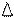Chapter 10.3, Problem 30E### Elementary Geometry for College St...

6th Edition
Daniel C. Alexander + 1 other
ISBN: 9781285195698

#### Solutions

Chapter
Section### Elementary Geometry for College St...

6th Edition
Daniel C. Alexander + 1 other
ISBN: 9781285195698
Textbook Problem
35 views

#The drawing shows parallelogram R S T V .a) What type of number is r ?b) Find an expression for R S .c) Describe the coordinate t in terms of the other variables shown.To determine

(a)

To find:

The type of number r using the given parallelogram.

Explanation

The given figure is shown below.

To determine

(b)

To find:

The expression for RS.

To determine

(c)

To describe:

The variable t in terms of other variables.

### Still sussing out bartleby?

Check out a sample textbook solution.

See a sample solution

#### The Solution to Your Study Problems

Bartleby provides explanations to thousands of textbook problems written by our experts, many with advanced degrees!

Get Started

#### Find more solutions based on key concepts